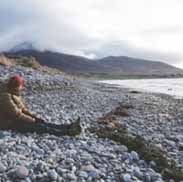# Inorganic Chemistry Final Exam ReviewTony Foust
question

chemistry

the study of the composition, structure, and properties of matter; the processes that matter undergoes; and the energy changes that accompany these processes
question

extensive properties

depend on the amount of matter that is present (volume, mass)
question

intensive properties

do not depend on the amount of matter present (melting point, boiling point, density)
question

solid

has definite volume and definite shape
question

liquid

has definite volume but indefinite shape
question

gas

has neither definite volume nor definite shape
question

plasma

high temperature state in which atoms lose most of their electrons
question

homogenous

uniform in composition (water)
question

heterogenous

not uniform in composition (pulpy orange juice)
question

Law of Conservation of Mass

Matter cannot be created or destroyed.
question

pure substance

a substance that cannot be separated without changing its identity
question

kg

SI unit of mass
question

l

SI unit of volume
question

m

SI unit of length
question

mole

SI unit for the amount of a substance
question

density

mass/volume
question

accuracy

refers to the closeness of measurements to the correct or accepted value of the quantity measured
question

precision

refers to the closeness of a set of measurements of the same quantity made in the same way
question

percentage error

question

deci

1/10
question

centi

1/100
question

milli

1/1000
question

Law of Definite Proportions

the fact that a chemical compound contains the same elements in exactly the same proportions by mass regardless of the size of the sample or source of the compound
question

Dalton

-solid, sphere, simplistic -did not have a nucleus, neutrons, protons, and electrons
question

Thomson

-plum pudding model -electrons floated around aimlessly -positive particles floating too
question

Rutherford

-planetary model -atom has a tiny, heavy, nucleus surrounded by lighter negatively charged electrons -Gold Foil experiment -protons were in center nucleus
question

Bohr

-electrons travel around the nucleus in circular orbits -quantum model -electron cloud with orbitals
question

isotope

atoms of the same element that have different masses (a nuclide)
question

6.02 * 10^23
question

a form of energy that exhibits wavelike behavior as it travels through space
question

wavelength

(λ) the distance between the crests of two waves
question

frequency

(v) the number of waves that pass a given point in a specific time, usually one second ex. Hz
question

photoelectric effect

refers to the emission of electrons from a metal when light shines on the metal
question

quantum

the minimum quantity of energy that can be lost or gained by an atom
question

orbital

a three-dimensional region around the nucleus that indicates the probable location of an electron
question

quantum numbers

specify the properties of atomic orbitals and the properties of electrons in orbitals
question

Planck’s constant

6.626 * 10^-34
question

speed of light (λ*v)

3.0 * 10^8
question

electromagnetic spectrum

question

orbital quantum number

s,p,d,f
question

principal quantum number

1-7 energy levels
question

Aufbau principle

an electron occupies the lowest-energy orbital that can receive it
question

Hund’s rule